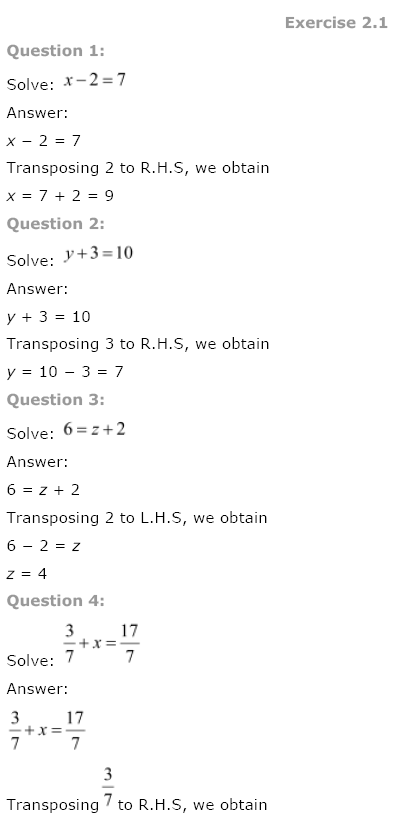## NCERT Solutions for Class 8th Maths Chapter 2 Linear Equations in One Variable

National Council of Educational Research and Training (NCERT) Book Solutions for Class 8th
Subject: Maths
Chapter: Chapter 2 – Linear Equations in One Variable

Class 8th Maths Chapter 2 Linear Equations in One Variable NCERT Solution is given below.« Previous Next »